## Sunday, February 6, 2011

### Geographic Coordinates Systems

This is something I believe every GIS Programmer is supposed to know.

A few weeks ago I had some problem with Union of IPolyline into one IPolyline and someone who is from the company that provides support for ESRI products in our region tried to help me. We were in the middle of debugging and he noticed that the length of one of the original IPolline was something like 0.000001. He thought that the problem was that my line was too small – less than a CM long.

I was shocked that he could tell the length of the line, but then he explained himself and said that 0.01 was a CM – and then I was shocked for a different reason…

I knew where he got it wrong. He was used to working with a coordinate system like UTM WGS84 where the length of the lines is in meters. But I was working with GEO WGS84 where the coordinates are in degrees and the length of the lines is NOT in meters.

I believe it is very similar to the old mistake NASA once made where some of their spacecraft parts were made in CM and some in inches (see CNN: Metric mishap caused loss of NASA orbiter). At that time that mistake cost 125 million dollars. Mistakes in Geographic Coordinate can cost lives in a military assault where accurate coordinates are essential.

### GEO Coordinates

GEO Coordinate (called also GCM=Geographic Coordinate System) use degrees of latitude and longitude to describe a location on the earth’s surface. The latitude lines are parallel to the the equator (between the North and South poles), The North pole has 90° and the South pool has -90° (the equator has 0°).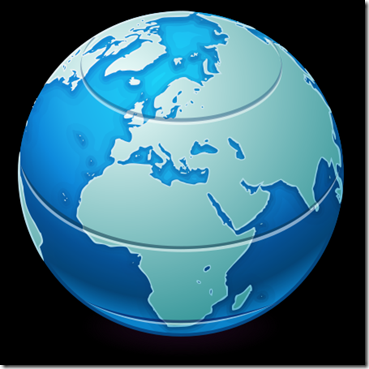(Not mine taken from Wikipedia)

The longitude lines run from the North pole to the South pole, only at the equator the distance between the lines is equal to the distance between the latitude lines. The degrees for the lines run from 0° to 180° for East or West (or 360° all around). The 0° line passes Greenwich, England.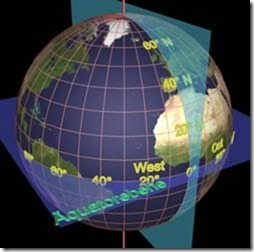(Not mine from here)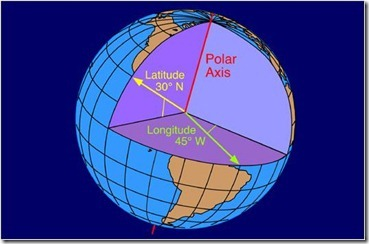(Not mine from here)

### UTM Coordinates

UTM Coordinates (Universal Transverse Mercator) on the other hand is a metric system that project the Earth’s spherical surface onto a two-dimensional plane. In one way of this system the globe is divided to 1200 tiles (maps in an atlas usually use this way of the system):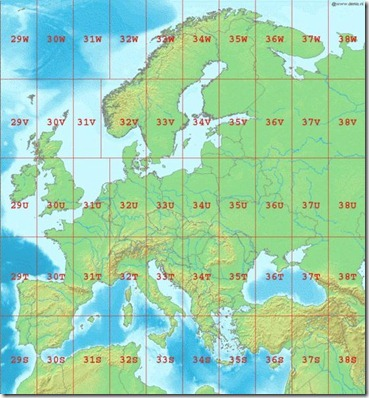(Not mine from here)

In the other way the globe is divided to 60 zones (each zone has 6°) which are divided further to North and South (ESRI uses this way of the system):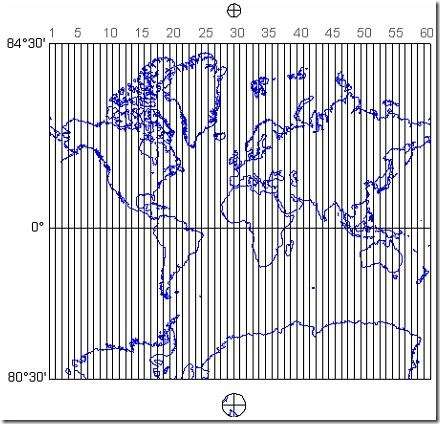(Not mine from here)

Both this ways were devises because the metric distance in them is optimal

This is in Datum WGS84 you should be aware that there are other Datums.

When calculating a distance in UTM where the coordinates are not in the same tile the distance should be calculated to the border of the tile and then continue through the tiles until reaching the second point: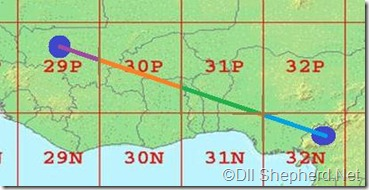(Every different line in this drawing should be calculated independently)

### So what’s the difference?

• A distance between point in UTM (in the same tile) will be in meters, where as in GEO the distance is not as accurate since the degrees of longitude lines does not equal everywhere on earth.
• A GEO coordinate of 61.44, 25.40 will be presented in UTM as 35V 414668 6812844.

### How do I use the coordinate systems in code?

In arcObjects the coordinate systems are called Spatial References and are set in a property named SpatialReference in IGeometry.

GEO WGS84 Spatial Reference(one of the useful methods I have on my team’s application):

1. public static ISpatialReference CreateWgsSpatialReference()
2. {
3.     SpatialReferenceEnvironment spatialReferenceEnvironment = new SpatialReferenceEnvironmentClass();
4.     ISpatialReference wgs84SpatialReference =
5.         spatialReferenceEnvironment.CreateGeographicCoordinateSystem((int)esriSRGeoCSType.esriSRGeoCS_WGS1984);
6.     return wgs84SpatialReference;
7. }

UTM WGS84 Spatial Reference for zone 14N:

1. SpatialReferenceEnvironment spatialReferenceEnvironment = new SpatialReferenceEnvironmentClass();
2.  ISpatialReference wgs84UtmSpatialReference =
3.      spatialReferenceEnvironment.CreateProjectedCoordinateSystem((int)esriSRProjCSType.esriSRProjCS_WGS1984UTM_14N);

You should note that there are many such spatial references in UTM WGS84, for example:

1. esriSRProjCS_WGS1984UTM_55N = 32655,
2. esriSRProjCS_WGS1984UTM_56N = 32656,
3. esriSRProjCS_WGS1984UTM_57N = 32657,
4. esriSRProjCS_WGS1984UTM_58N = 32658,
5. esriSRProjCS_WGS1984UTM_59N = 32659,
6. esriSRProjCS_WGS1984UTM_60N = 32660,
7. esriSRProjCS_WGS1984UTM_1S = 32701,
8. esriSRProjCS_WGS1984UTM_2S = 32702,
9. esriSRProjCS_WGS1984UTM_3S = 32703,
10. esriSRProjCS_WGS1984UTM_4S = 32704,

(Taken from ESRI reflected code)

A method that creates a point with GEO WGS84 coordinate system:

1. public static IPoint CreatePoint(double x, double y)
2. {
3.     IPoint point = new PointClass { X = x, Y = y, SpatialReference = CreateWgsSpatialReference() };
4.
5.     return point;
6. }

I would like to thank Asafi Yosef (by far the best Geodesist that I know) for reviewing this post and correcting some of my mistakes.

Edit 27/03/2011: Found this nice link that show the distortions caused by different Coordinate Systems. If you want to see why GEO WGS84 is bad for distances then check it out.

Resources:

Tissot's indicatrix

Keywords: ESRI, Coordinate system, Coordinate, Point, WGS84, GEO, UTM, IPoint, distance